# UTF-8 processing using SIMD (SSE4)

SIMD: "Single instruction, multiple data" is a class of instructions present in many CPUs today. For example, on the Intel CPU they are known under the SSE acronym. Those instructions enable more parallelism by operating simultaneously on multiple data.

In this blog post I will present a method for converting UTF-8 text to UTF-16 using SSE4 compiler intrinsics. My goal is also to introduce you to the SIMD intrinsics, if you are not familiar with them yet.

CPUs can parallelize computations by using specials instructions that operate simultaneously on multiples values. There are large registers that contain vectors of values. For example, the SSE registers are 128 bits vectors that can contain either 2 double, 4 float, 4 int, 8 short or up to 16 char values. Then, one single instruction can add, substract, multiply, ... all those values and put the result in another vector register.
This is called SIMD for Single Instruction Multiple Data.

Compilers can sometimes automatically vectorize the contents of a simple loop, but they are not really good at it. If the loop is too complex or if it depends on the previous iteration, the compiler will fail to vectorize. (Even if it would be obvious for an human). The vectorization therefore has to be done manually.

This is where compiler intrinsics help us. They are special functions handled directly in the compiler who will emit the instruction we want.

The problem with intrinsics is that they are not really portable. They target a specific architecture. This means you need to write your algorithms for the different CPU architectures you target. Fortunately, the main compilers use the same intrinsics so they are portable across compilers and operating systems.

You are supposed to detect the support of the instruction set at run-time. What is usually done is to write several variants of the function for the different target architecture and have a function pointer that is set to the best variant for the running architecture.

The best documentation I could find is the reference on MSDN. You will have to browse it a bit to see what the available intrinsics are.

## The Task: Converting UTF-8 to UTF-16

I got interested in SIMD when Benjamin was using them to speed up QString::fromLatin1, which converts a `char*` encoded in Latin-1 to UTF-16 (QString's internal encoding). After he explained to me how it works, I was able to speed up some of the drawing code inside Qt.
However in Qt5, we changed the default string encoding from Latin-1 to UTF-8.

UTF-8 can represent all of Unicode while Latin-1 can only represent the alphabet of a few latin based languages. UTF-8 parsing is much more difficult to vectorize because a single code point can be encoded by a variable number of bytes.

This table explains the correspondence between the bits in UTF-8 and UTF-16:

UTF-8UTF-16
ASCII (1 byte) `0aaaaaaa` `00000000 0aaaaaaa`
Basic Multilingual Plane
(2 or 3 bytes)
```110bbbbb 10aaaaaa``` ```00000bbb bbaaaaaa```
```1110cccc 10bbbbbb 10aaaaaa``` ```ccccbbbb bbaaaaaa```
Supplementary Planes
(4 bytes)
```11110ddd 10ddcccc 10bbbbbb 10aaaaaa```
`uuuu = ddddd - 1`
```110110uu uuccccbb 110111bb bbaaaaaa```

I was wondering if we could improve QString::fromUtf8 using SIMD.
Most of the strings are just ASCII which is easy to vectorize, but I wanted to also try to go ahead and vectorize the more complicated cases as well.
However, the 4 bytes sequences (Supplementary Planes) are very rarely used (most used languages and useful symbols are already in the Basic Multilingual Plane) and also more complicated so I did not vectorize that case for the scope of this blog post. This is left as an exercise for the reader ;-)

## Previous work

UTF-8 processing is a very common task. I was expecting that the problem would be already solved. Currently however most libraries and applications still have a scalar implementation. Few use vectorization, but only for ASCII.

There is a library called u8u16. It uses a clever technique by separating the bits in individual vectors. It is processing 128 char at the time in 8 different vectors.
I wanted to try a different approach and work directly on one vector. Working with 16 char at the time allows speeding up the processing of smaller strings.

## The Easy Case: ASCII

I'm going to start by explaining the simple case when we only have ASCII. This is very easy to vectorize because for ASCII one byte in UTF-8 is always one byte in UTF-16 with `\0` bytes in between.
ASCII is also a very common encoding so it make sense to have a special case for it.

I will start by showing the code and then explaining it

Our function takes a pointer `src` to the UTF-8 buffer of length `len`, and a pointer `dst` to a buffer of size at least `len*2` where we will store the UTF-16 output. The function returns the size of the UTF-16 output.

```int fromUtf8(const char *src, int len, unsigned short *dst) {
/* We will process the input 16 bytes at a time,
so the length must be at least 16. */
while(len >= 16) {

/* Load 128 bit into a vector register. We use the 'loadu' intrinsic
but here we don't know if the source is aligned */

/* Detect if it is ASCII by looking if one byte has the high bit set. */

// unpack the first 8 bytes, padding with zeros
__m128i firstHalf = _mm_unpacklo_epi8(chunk, _mm_set1_epi8(0));
// and store to the destination
_mm_storeu_si128(reinterpret_cast<__m128i*>(dst), firstHalf);

// do the same with the last 8 bytes
__m128i secondHalf = _mm_unpackhi_epi8 (chunk, _mm_set1_epi8(0));
_mm_storeu_si128(reinterpret_cast<__m128i*>(dst+8), secondHalf);

dst += 16;
src += 16;
len -= 16;
continue;
}

// handle the more complicated case when the chunk contains multi-bytes
// ...
}
// handle the few remaining bytes using a classical serial algorith
// ...
}
```

The type `__m128i` represents a vector of 128 bits. It is most likely going to be stored in a SSE register.
Since we don't know the alignment, we load the data with `_mm_loadu_si128`. There is also the `_mm_load_si128` that assumes the memory is aligned on 16 bytes. Because it is faster to operate on aligned data, some algorithms sometimes start with a prologue to handle the first bytes until the rest is aligned. I did not do that here but we could optimize the ASCII processing further by doing so.

Once we have loaded 16 bytes of data into `chunk`, we need to check if it only contains ASCII. Each ASCII byte should have its most significant bit set to 0. `_mm_movemask_epi8` creates a 16bit mask out of most significant bits of our 16 bytes in the vector. If that mask is 0 we only have ASCII in that vector.

The next operation is to expand with zeroes. We will do what is called unpacking. The instructions `_mm_unpackhi_epi8` and `_mm_unpacklo_epi8` are going to interleave two vectors. Because the result of an unpack is on 32 bytes, there is two operations: one to get the first 16 high bytes, and the other to get the low bytes.
`_mm_set1_epi8(0)` creates a vector filled with zeroes.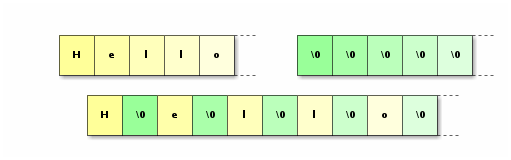Note that vectors are in little endian, so I show the least significant bytes first

Now, we can just store the result with `_mm_storeu_si128`. We use the unaligned operation again.

## General Algorithm

Now that I have introduced you to the ASCII decoding process, we can move on to the general case, in which we might have multiple bytes for one character.

The algorithm proceeds with the following steps:

1. First, we will classify each bytes so we know where the values in the sequence are and how long they are. This information will be stored in vectors that we can use to mask so operations we do later operate only on a certain category of bytes.
2. Then, we will operate on the data to to find the actual Unicode value behind each character. They will be stored at the location of the last byte of a sequence, into two different vectors: one for the low bits and one for the high bits.
3. Then we need to shuffle the vectors to remove the possible "gaps" left by multi-bytes sequences.
4. Finally, we can store the result and advance the pointers.

I will explain every step in more detail.

In our example, I will decode the string x(α+β)²γ², which is represented in UTF-8 as `x\e2\89\a4(\ce\b1+\ce\b2)\c2\b2\ce\b3\c2\b2`. The "≤" is represented by 3 bytes, the Greek letters by two bytes, and the "²" also by two bytes. The total length is 17 bytes, so the last byte will be truncated when loaded into the vector

## Classification

At the end of this step, we will have the following vectors:

• `mask` tells which bit needs to be set to 0 in order to only keep the bits that are going to end up in the Unicode value. Bits of mask that are set will be unset from the data.
• `count` is zero if the corresponding byte is ASCII or a continuation byte. If the corresponding byte is starting a sequence, then it is the number of bytes in that sequence.
• `counts` represents (for each byte) how many bytes are remaining in this sequence. It is 0 for ASCII, 1 for the last byte in a sequence, and so on.

We start by finding `count` and `mask`. I do that by computing a vector that I called `state`, in which the 5 higher bits are the mask and the 3 lower bits represent the count.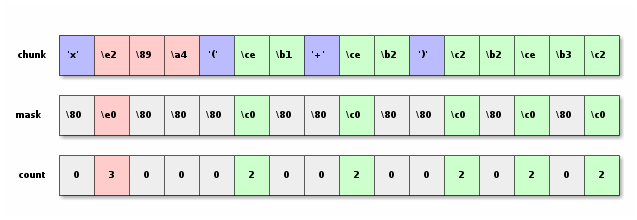We will use the `_mm_cmplt_epi8` instruction. It compares two vectors of 16 signed char, and returns a mask vector where each byte is equal to `0xff` or `0x00` depending of the result of the comparison between the corresponding chars.
We can then use this mask as input to the `_mm_blendv_epi8` SSE4 instruction. It takes 3 input arguments: two vectors and a mask. It returns a vector that has the bytes of the second input where the most significant bit of the corresponding byte of the mask is one and the bytes of the first input otherwise. In other words, for each 16 bytes of the vectors, we have:
`output[i] = mask[i] & 0x80 ? input2[i] : input1[i]`

The problem of `_mm_cmplt_epi8` is that it only works on signed bytes. That is why we add `0x80` to everything simulate unsigned comparison.

```    // The state for ascii or contiuation bit: mask the most significant bit.
__m128i state = _mm_set1_epi8(0x0 | 0x80);

// Add an offset of 0x80 to work around the fact that we don't have
// unsigned comparison

// If the bytes are greater or equal than 0xc0, we have the start of a
// multi-bytes sequence of at least 2.
// We use 0xc2 for error detection, see later.
__m128i cond2 = _mm_cmplt_epi8( _mm_set1_epi8(0xc2-1 -0x80), chunk_signed);

state = _mm_blendv_epi8(state , _mm_set1_epi8(0x2 | 0xc0),  cond2);

__m128i cond3 = _mm_cmplt_epi8( _mm_set1_epi8(0xe0-1 -0x80), chunk_signed);

// We could optimize the case when there is no sequence logner than 2 bytes.
// But i did not do it in this version.
//if (!_mm_movemask_epi8(cond3)) { /* process max 2 bytes sequences */ }

state = _mm_blendv_epi8(state , _mm_set1_epi8(0x3 | 0xe0),  cond3);

// In this version I do not handle sequences of 4 bytes. So if there is one
// we break and do a classic processing byte per byte.
__m128i cond4 = _mm_cmplt_epi8(_mm_set1_epi8(0xf0-1 -0x80), chunk_signed);

// separate the count and mask from the state vector
__m128i count =  _mm_and_si128(state, _mm_set1_epi8(0x7));

```

From `count` I will be able to compute `counts`. I will use `_mm_subs_epu8` which substracts two vector of unsigned bytes and saturate (so if you underflow, it sets 0). Then I shift that result, and add it to the count. Then we do the same, but we substract 2 and shift the result two bytes.

`_mm_slli_si128` shifts a vector on the left by the given number of bytes. Notice again that we work on little endian. So that is why I shifted right on the picture, but left in the code.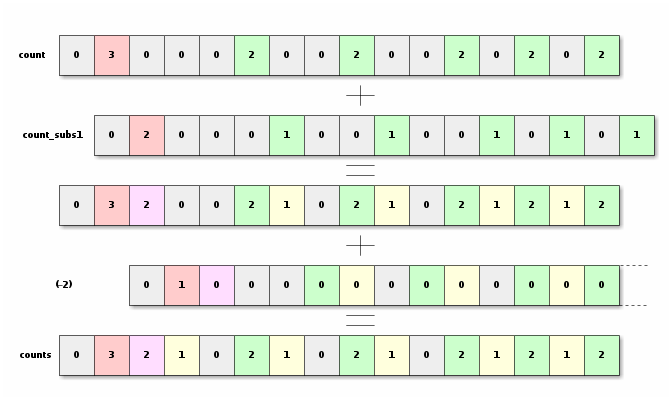```    //substract 1, shift 1 byte and add
__m128i count_subs1 = _mm_subs_epu8(count, _mm_set1_epi8(0x1));
__m128i counts = _mm_add_epi8(count, _mm_slli_si128(count_subs1, 1));

//substract 2, shift 2 bytes and add
_mm_subs_epu8(counts, _mm_set1_epi8(0x2)), 2));
```

## Processing

The goal of this step is to end up with the final unicode bytes values. They will be split into two vectors (one for the high bytes and one for the low bytes). We keep them in the location of the last byte of a sequence, which means that there will be "gaps" in the vector.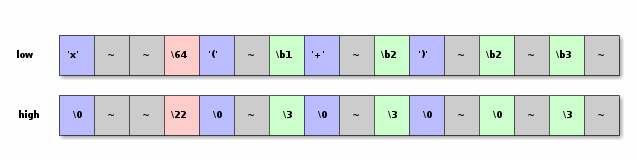There is no instructions that shift the whole vector by an arbitrary number of bits. It is only possible to shift by an integer number of bytes with `_mm_slli_si128` or `_mm_srli_si128`. The instructions that shift by an abitrary number of bits only work on vector with elements of 16 or 32 bits. No 8 bits elements. We will use what we have and will first shift by one byte (in `chunk_right`) and then shift and mask to get what we want.

```    // Mask away our control bits with ~mask (and not)
// from now on, we only have usefull bits

// shift by one byte on the left
__m128i chunk_right = _mm_slli_si128(chunk, 1);

// If counts == 1,  compute the low byte using 2 bits from chunk_right
__m128i chunk_low = _mm_blendv_epi8(chunk,
_mm_or_si128(chunk, _mm_and_si128(
_mm_slli_epi16(chunk_right, 6), _mm_set1_epi8(0xc0))),
_mm_cmpeq_epi8(counts, _mm_set1_epi8(1)) );

// in chunk_high, only keep the bits if counts == 2
__m128i chunk_high = _mm_and_si128(chunk ,
_mm_cmpeq_epi8(counts, _mm_set1_epi8(2)));
// and shift that by 2 bits on the right.
// (no unwanted bits are comming because of the previous mask)
chunk_high = _mm_srli_epi32(chunk_high, 2);

// Add the bits from the bytes for which counts == 3
__m128i mask3 = _mm_slli_si128(cond3, 1); //re-use cond3 (shifted)
chunk_high = _mm_or_si128(chunk_high, _mm_and_si128(
_mm_and_si128(_mm_slli_epi32(chunk_right, 4), _mm_set1_epi8(0xf0)),
```

## Shuffle

Now, we need to get rid of the gaps in our strings. We will start by computing, for each byte, the number of bytes we need to shift. We notice that `count_subs1`is the number of bytes we need to skip for a given sequence. We will then accumulate all those number in order to get the number of bytes we need to shift each bytes. Then we reset to zero all the bytes that are supposed to go away.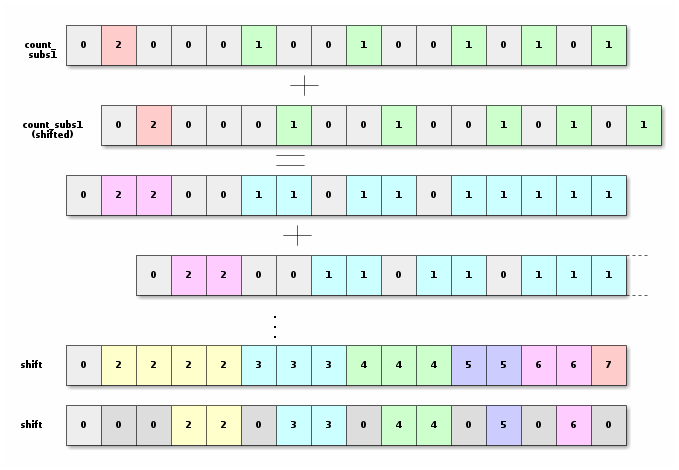```    __m128i shifts = count_subs1;

// keep only if the corresponding byte should stay
// that is, if counts is 1 or 0  (so < 2)
shifts  = _mm_and_si128 (shifts , _mm_cmplt_epi8(counts, _mm_set1_epi8(2)));
```

We will shift the `shift` vector so the meaning of it becomes the number of bytes that are separating a byte in its final location from the byte in its original location.

The maximum we can shift is 10 (if everything is a 3 bytes sequence). We can then compute in 4 steps.
We will shift the interesting bit of the vector `shift`, so it can be used to control the blend operation.

```
shifts = _mm_blendv_epi8(shifts, _mm_srli_si128(shifts, 1),
_mm_srli_si128(_mm_slli_epi16(shifts, 7) , 1));

shifts = _mm_blendv_epi8(shifts, _mm_srli_si128(shifts, 2),
_mm_srli_si128(_mm_slli_epi16(shifts, 6) , 2));

shifts = _mm_blendv_epi8(shifts, _mm_srli_si128(shifts, 4),
_mm_srli_si128(_mm_slli_epi16(shifts, 5) , 4));

shifts = _mm_blendv_epi8(shifts, _mm_srli_si128(shifts, 8),
_mm_srli_si128(_mm_slli_epi16(shifts, 4) , 8));

```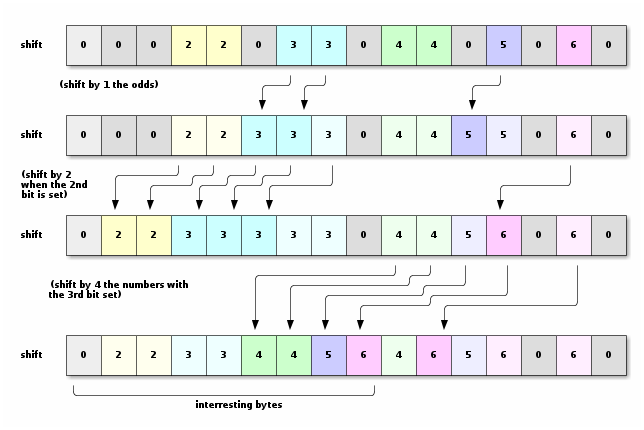Then we can then know, for each byte in the final vector, its location in the original vector. With that information, we can use `_mm_shuffle_epi8` (SSSE3) to shuffle the vector and remove the "gaps". Then we can unpack the result and store it, and we are almost done.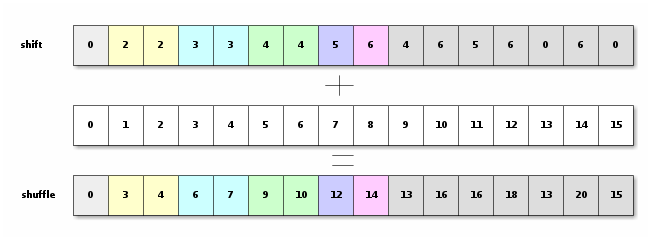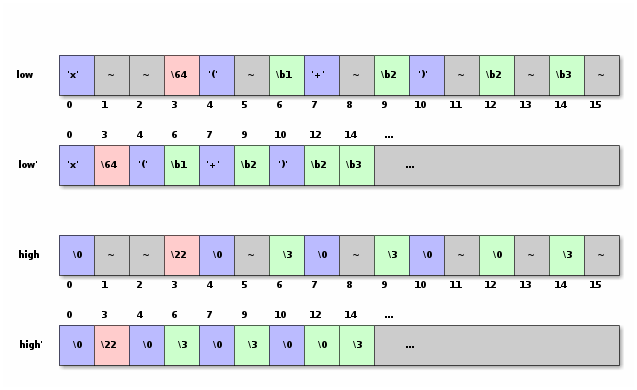```    __m128i shuf = _mm_add_epi8(shifts,
_mm_set_epi8(15,14,13,12,11,10,9,8,7,6,5,4,3,2,1,0));

// Remove the gaps by shuffling
shuffled_low = _mm_shuffle_epi8(chunk_low, shuf);
shuffled_high = _mm_shuffle_epi8(chunk_high, shuf);

// Now we can unpack and store
__m128i utf16_low = _mm_unpacklo_epi8(shuffled_low, shuffled_high);
__m128i utf16_high = _mm_unpackhi_epi8(shuffled_low, shuffled_high);
_mm_storeu_si128(reinterpret_cast<__m128i*>(dst), utf16_low);
_mm_storeu_si128(reinterpret_cast<__m128i*>(dst+8) , utf16_high);
```

## Error Detection

So far we have only considered valid UTF-8. But a conform UTF-8 decoder should also handle broken UTF-8. It should detect the following error cases:

1. Not enough, misplaced or too many continuation characters.
2. Overlong forms: If a sequence decodes to a value that could have been encoded in a shorter sequence. For example the quote must be encoded in its ASCII form (`\22`) and not in a multi-byte sequence (`\c0\a2` or `\e0\80\a2`). Not forbidding those forms could lead to security problems, e.g. if the the quote where escaped in the utf-8 input string, but appears again later when decoded.
3. Invalid or reserved Unicode code point (for example, the one reserved for UTF-16 surrogates)

Since error is not the common case, what I do in that case is to break, and let the rest be handled by the sequential algorithm (Just like when we have 4 bytes sequences).

```
// ASCII characters (and only them) should have the
// corresponding byte of counts equal 0.
break;

// The difference between a byte in counts and the next one should be negative,
// zero, or one. Any other value means there is not enough continuation bytes.
counts), _mm_set1_epi8(1))))
break;

// For the 3 bytes sequences we check the high byte to prevent
// the over long sequence (0x00-0x07) or the UTF-16 surrogate (0xd8-0xdf)
__m128i high_bits = _mm_and_si128(chunk_high, _mm_set1_epi8(0xf8));
_mm_or_si128(_mm_cmpeq_epi8(high_bits,_mm_set1_epi8(0x00)) ,
_mm_cmpeq_epi8(high_bits,_mm_set1_epi8(0xd8))) ))
break;

// Check for a few more invalid unicode using range comparison and _mm_cmpestrc
const int check_mode = _SIDD_UWORD_OPS | _SIDD_CMP_RANGES;
if (_mm_cmpestrc( _mm_cvtsi64_si128(0xfdeffdd0fffffffe), 4,
utf16_high, 8, check_mode) |
_mm_cmpestrc( _mm_cvtsi64_si128(0xfdeffdd0fffffffe), 4,
utf16_low, 8, check_mode))
break;
```

Now we are almost done. We just need to advance the source and destination pointers. The source pointer usually advance by 16, unless it ends in the middle of a sequence. In that case, we need to roll back 1 or 2 chars. For that, we look at the end of the `counts` vector.
Then we can see how much we need to advance the destination by looking at the end of the shift vector.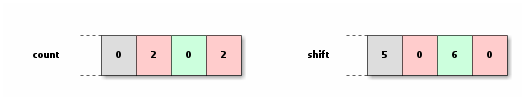```    int s = _mm_extract_epi32(shifts, 3);

int c = _mm_extract_epi16(counts, 7);
int source_advance = !(c & 0x0200) ? 16 : !(c & 0x02) ? 15 : 14;

```

## Putting it all together

Now we are done, you can see the result there

I mixed all the operations to limit the dependencies between the operations that are next to each other to achieve better pipeline performance. I am surprised that the compiler did not do that for me.

## Benchmarks Results

Long Chinese text (8KiB)36 bytes of chinese text Long ASCII (24KiB) 20 ASCII bytes
Iconv 62000ns 380ns 165000ns 230ns
Qt (scalar) 43000ns 180ns 55000ns 67ns
u8u16 20000ns 300ns 8000ns 37ns
This solution (SSE4.1) 22000ns110ns 15000ns 40ns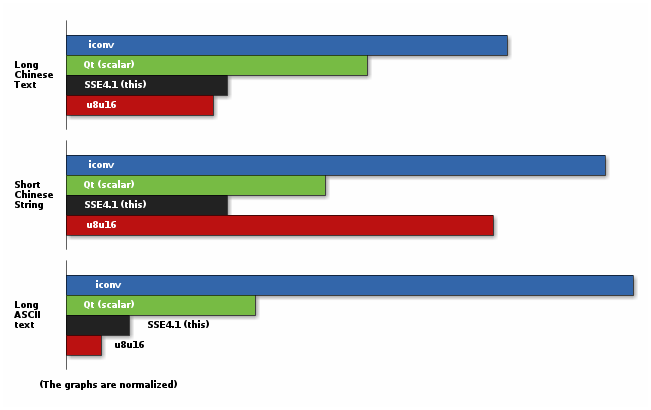We beat u8u16 on small strings but it is better on large strings because it processes more bytes at the same time. We are still better than any scalar processing.

I added some debug output in the implementation of `QString::fromUtf8` in Qt 4.8 and ran few KDE applications translated in French. The average size of strings that goes through this function is 9 characters. More than 50% of the string are less than 2 character long, 85% are less than 16, and 13% are between 16 and 128 characters long. 98% of the strings where only composed of ASCII. The rest was mainly having 2 bytes sequences and very few 3 bytes sequences. There is no sequence of 4 bytes.
Also consider that the processing of UTF-8 is probably far from being the bottleneck of any application that does something useful.

So was my exercise useless? Maybe in practice, but I think there is still something to learn from this that can be applied to another algorithm that might be a bottleneck.

### Article posted by Olivier Goffart on 26 July 2012

Load Comments...